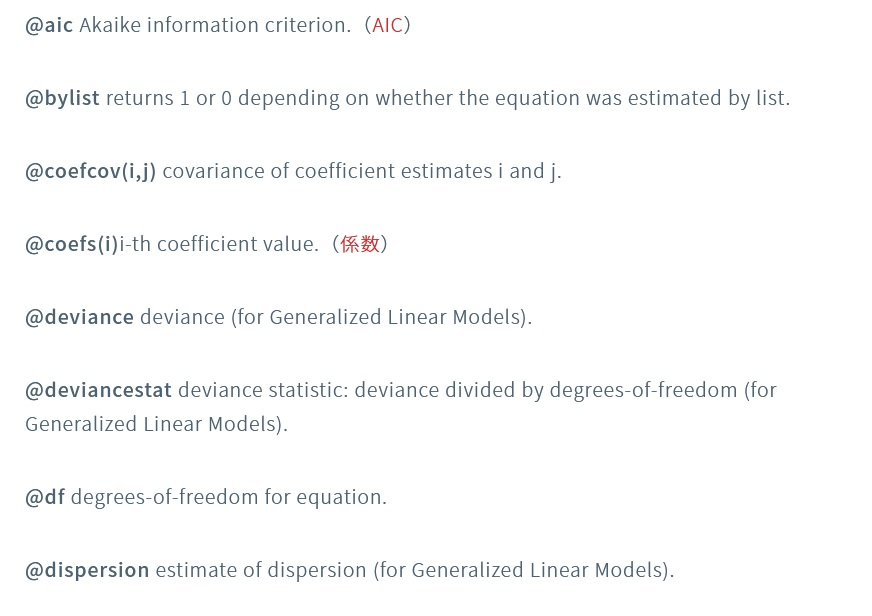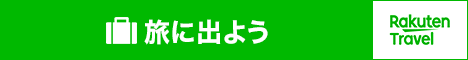Eviews

# 【EViews】推計結果の保存｜保存できるものをすべて紹介この記事ではそれらをすべて載せて、プログラムを書く際の参考にしていただきたいと思います。【EViews】EViewsのまとめ 経済統計の使い方では、統計データの入手法から分析法まで解説しています。 https://officekaisuiyoku.com...## 方程式の推計

EViewsで方程式の係数を推定し、係数などを保存するには以下の手続きが必要です。

table tab
equation 方程式名

tab(1,1)=方程式名.@coefs(1)

## 方程式のデータメンバー

### Scalar Values（数値）

@aic Akaike information criterion.（AIC

@bylist returns 1 or 0 depending on whether the equation was estimated by list.

@coefcov(i,j) covariance of coefficient estimates i and j.

@coefs(i)i-th coefficient value.（係数

@deviance deviance (for Generalized Linear Models).

@deviancestat deviance statistic: deviance divided by degrees-of-freedom (for Generalized Linear Models).

@df degrees-of-freedom for equation.

@dispersion estimate of dispersion (for Generalized Linear Models).

@dw Durbin-Watson statistic.（ダービンワトソン比

@fF-statistic.（F値）

@finalbw returns the final bandwidth used in functional coefficient estimation.

@fixeddisp indicator for whether the dispersion is a fixed value (for Generalized Linear Models).

@fprob probability value of the F-statistic.（F検定のp値

@hacbw bandwidth for HAC estimation of GMM weighting matrix or long-run covariance in cointegrating regression (if applicable).

@hq Hannan-Quinn information criterion.

@instrank rank of instruments (if applicable).

@jstatJ-statistic — value of the GMM objective function (for GMM and TSLS).

@jprob probability value of the J-statistic.

@lambdamin minimum lambda value from ENET cross-validation.

@logl value of the log likelihood function.

@lrprob probability value of likelihood ratio statistic (if applicable).

@lrstat likelihood ratio statistic (if applicable).

@lrvar long-run variance estimate for cointegrating regression (if applicable).

@meandep mean of the dependent variable.

@nbreaks number of breaks in breakpoint least squares and thresholds in threshold regression.

@ncases number of cases.

@nclusters number of clusters used in computing cluster robust covariances.

@ncoef number of estimated coefficients.

@ncross number of cross-sections used in estimation (equal to 1 for non-panel workfiles).

@npers number of workfile periods used in estimation (same as @regobs for non-panel workfiles).

@nregimes number of regimes in a switching and breakpoint regression.

@nthresholds number of thresholds in threshold regression.

@ntreatment difference-in-difference number of cross sections receiving treatment.

@objective quasi-likelihood objective function (if applicable).

@pearsonssr Pearson sum-of-squared residuals (for Generalized Linear Models).

@pearsonstat Pearson statistic: Pearson SSR divided by degrees-of-freedom (for Generalized Linear Models).

@pilotbw returns the pilot bandwidth used in functional coefficient estimation.

@qlrprob probability value of quasi-likelihood ratio statistic (if applicable).

@qlrstat quasi-likelihood ratio statistic (if applicable).

@quantdep quantile of dependent variable (for quantile regression).

@r2 R-squared statistic.

@rdeviance restricted (constant only) deviance (for Generalized Linear Models).

@regobs number of observations in regression.(サンプル数

@rlogl restricted (constant only) log-likelihood (if applicable).

@rmse root MSE.

@rn2 Rn-squared statistic.

@robf robust F-statistic (Wald-test form).

@robfprob robust F-statistic (Wald-test form) p-value.

@robjective restricted (constant only) quasi-likelihood objective function (if applicable).

@rw2 Rw-squared.

@schwarz Schwarz information criterion.

@sddep standard deviation of the dependent variable.

@se standard error of the regression.

@sparsity estimate of sparsity (for quantile regression).

@ssr sum of squared residuals.（誤差の二乗和

@ssr2 second-stage SSR.

@stderrs(i) standard error for coefficient i.（係数の標準誤差

@thresholds number of thresholds (for threshold regression).

@tstats(i)t-statistic or z-statistic value for coefficient i.（ｔ値

@wmeandep weighted mean of dependent variable (if applicable).

@wgtscale scaling factor for weights (if applicable).

c(i)i-th element of default coefficient vector for equation (if applicable).

### Vectors and Matrices（ベクトルと行列）

@ardlceccoefs returns a vector of coefficient estimates from the conditional error-correction (CEC) regression in univariate (N)ARDL estimation.

@ardleccoefs returns a vector of coefficient estimates from the traditional error-correction (EC) regression in univariate (N)ARDL estimation.

@ardlcoint returns a coef containing coefficients from the cointegrating relationship form of an ARDL estimation.

@ardllrcoefs returns a coef containing coefficients from the long run relationship form of a non-panel ARDL estimation.

@ardlsrcoefs returns a matrix where each row corresponds to an individual cross-section’s short-run coefficients. Only applicable for PMG/ARDL estimation.

@ardlsrses returns a matrix where each row corresponds to an individual cross-section’s short-run coefficient standard errors. Only applicable for PMG/ARDL estimation.

@coefcov covariance matrix for coefficient estimates.

@coefs coefficient vector.

@cvtrainindices training set indices for ENET cross-validation.

@cvtestindices test set indices for ENET cross-validation.

@effects vector of fixed and random effects estimates (if applicable).

@fcgrid returns a vector of unique grid values over which functional coefficients are evaluated in functional coefficient estimation.

@initprobs matrix containing initial probabilities for switching regression equations.

@lambda Elastic net lambda path.

@lambdacoefs Elastic net lambdas and coefficients.

@lambdaerrors Elastic net measurement error means and standard errors.

@lambdasum Elastic net summary path.

@pmgcxcoefs returns a matrix of coefficient estimates from the error-correction regressions for each cross-section in PMG estimation. Each column corresponds to a single cross-section and each column corresponds to a coefficient estimate from the traditional error-correction regression, in order of appearance.

@pmgcxses returns a matrix of coefficient standard error estimates from the error-correction regressions for each cross-section in PMG estimation. Each column corresponds to a single cross-section and each column corresponds to a coefficient standard error estimate from the traditional error-correction regression, in order of appearance.

@pmglrcoefs returns a vector of long-run (pooled) coefficient estimates in PMG estimation.

@pmgsrcoefs returns a vector of short-run (mean-group) coefficient estimates in PMG estimation.

@pvals vector containing the coefficient probability values.（p値のベクトル）

@stderrs vector of standard errors for coefficients.

@thresholds vector of threshold values for threshold estimation.

@tstats vector of t-statistic or z-statistic values for coefficients.

### String Values（文字列）

@ardlcointsubst returns string representation of the cointegration form of an ARDL equation with substituted coefficients.

@attr(“arg”) string containing the value of the arg attribute, where the argument is specified as a quoted string.

@breaks string representation of the breakpoints in breakpoint least squares or thresholds in threshold regression.

@coeflabels coefficient labels used in regression output table.

@coeflist returns a string containing a space delimited list of the coefficients used in estimation (e.g., “C(1) C(2) C(3)”). This function always returns the list of actual coefficients used, irrespective of whether the original equation was specified by list or by expression.

@command full command line form of the estimation command. Note this is a combination of @method, @options and @spec.

@depends string containing a list of the series in the current workfile on which this equation depends.

@description string containing the Equation object’s description (if available).

@detailedtype returns a string with the object type: “EQUATION”.

@displayname returns the equation’s display name. If the equation has no display name set, the name is returned.

@esteq returns string representation of the estimation equation.

@extralist space delimited list of the equation’s extra regressors. For equation’s estimated by ARCH, @extralist contains the variance equation terms. For equations estimated by CENSORED, this contains the error distribution terms. For all other equation methods it returns an empty string.

@instlist space delimited list of the equation instruments (if applicable).

@method command line form of estimation method (“ARCH”, “LS”, etc….).

@name returns the name of the Equation.

@options command line form of estimation options.

@remarks string containing the equation object’s remarks (if available).

@smpl description of the sample specified for estimation.

@spec original equation specification. Note this will be different from @varlist if the equation specification contains groups, or is specified by expression.

@subst returns string representation of the equation with substituted coefficients.

@type returns a string with the object type: “EQUATION”.

@updatetime returns a string representation of the time and date at which the equation was last updated.

@varlist space delimited list of the equation’s dependent variable and regressors if the equation was specified by list, or the equation’s underlying variables (both dependent and independent) if the equation was specified by expression.

@varselkept space delimited list of variables kept by model selection.

@varselrejected space delimited list of the variables dropped by model selection.